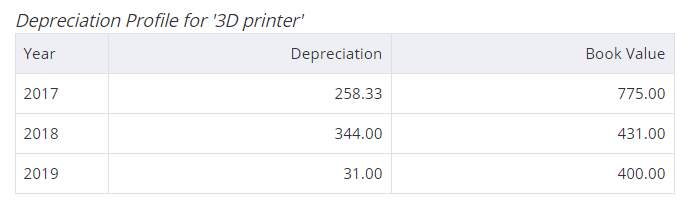Debitoor's accounting dictionary
Depreciation

# Depreciation – What is depreciation?

Depreciation is the permanent and continuing decrease in the quality, quantity or value of an asset.

Apply and track depreciation automatically over time with accounting and invoicing software like Debitoor. Try it free for 7 days.

Depreciation is the measure of wearing out of a fixed asset. All fixed assets are expected to be less efficient as time goes on and as they are continuously used in a business, for example.

Depreciation is calculated as the estimate of this measure of wear and is recorded in the Profit & Loss report either on a monthly or an annual basis. The cost of the asset less the total depreciation will give you the Net Book Value of the asset.

## Why depreciation is used

The idea of depreciation is to spread the cost of that capital asset over the period of its "useful life to the entity" that currently owns it.

If the full cost of the asset were to be borne in the year that it was purchased, then that year's expenditure would be unfairly penalised whilst expenditure during the remaining years, which were still receiving the benefit from the asset, would not be affected.

## How do assets depreciate?

Assets depreciate for two main reasons:

Wear and tear. For example, a car will decrease in value because of the mileage, wear on tyres, and other factors related to the use of the vehicle.

Obsolescence. Assets also decrease in value as they are replaced by newer models. Last year's car model is less valuable because there is a newer model in the marketplace.

## Depreciation calculation

Depreciation is calculated as follows: The original cost of the asset, minus the salvage value (the "scrap" value) then divided over the years of useful life of the asset.

For example: Jill’s design company purchases a 3D printer for £1,240, which they estimate that they will use for 3 years (the printer’s useful life). Under depreciation, the value of the printer decreases each year, with an estimated residual value at the end of the three years of: £400.

Straight-line depreciation (meaning the asset depreciates at the same rate over the years) then breaks down the value of the asset over the period of the 3 years:## Depreciation and Debitoor

When you add a new expense in Debitoor, you can select the option of whether to mark it as an asset. You will then be prompted to estimate the useful life of the asset (or how long it will be in your possession/use).

The purchase value and useful life are then used by Debitoor to automatically apply the most common form of depreciation methods: straight-line depreciation.